# Let a and b be elements of a group G such that b has order 2...

Let a and b be elements of a group G such that b has order 2 and ab=ba^-1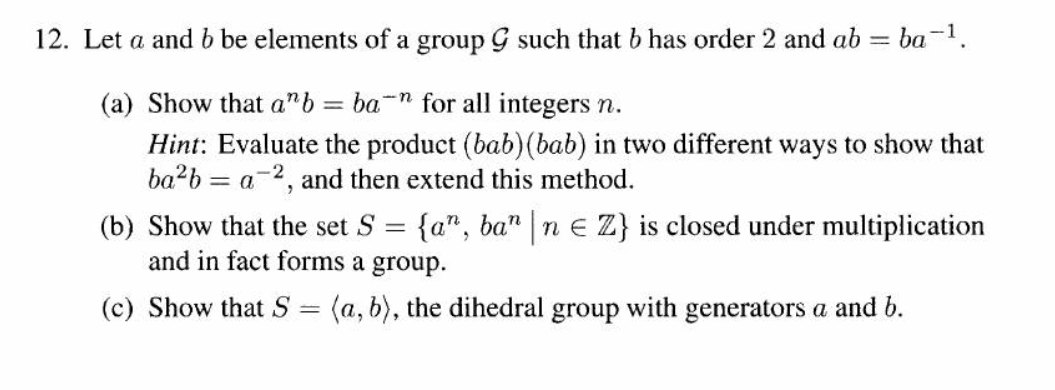12. Let a and b be elements of a group G such that b has order 2 and ab = ba-1. (a) Show that a” b = ba-n for all integers n. Hint: Evaluate the product (bab)(bab) in two different ways to show that ba+b = a-2, and then extend this method. (b) Show that the set S = {a”, ba" | n € Z} is closed under multiplication and in fact forms a group. (c) Show that S (a,b), the dihedral group with generators a and b.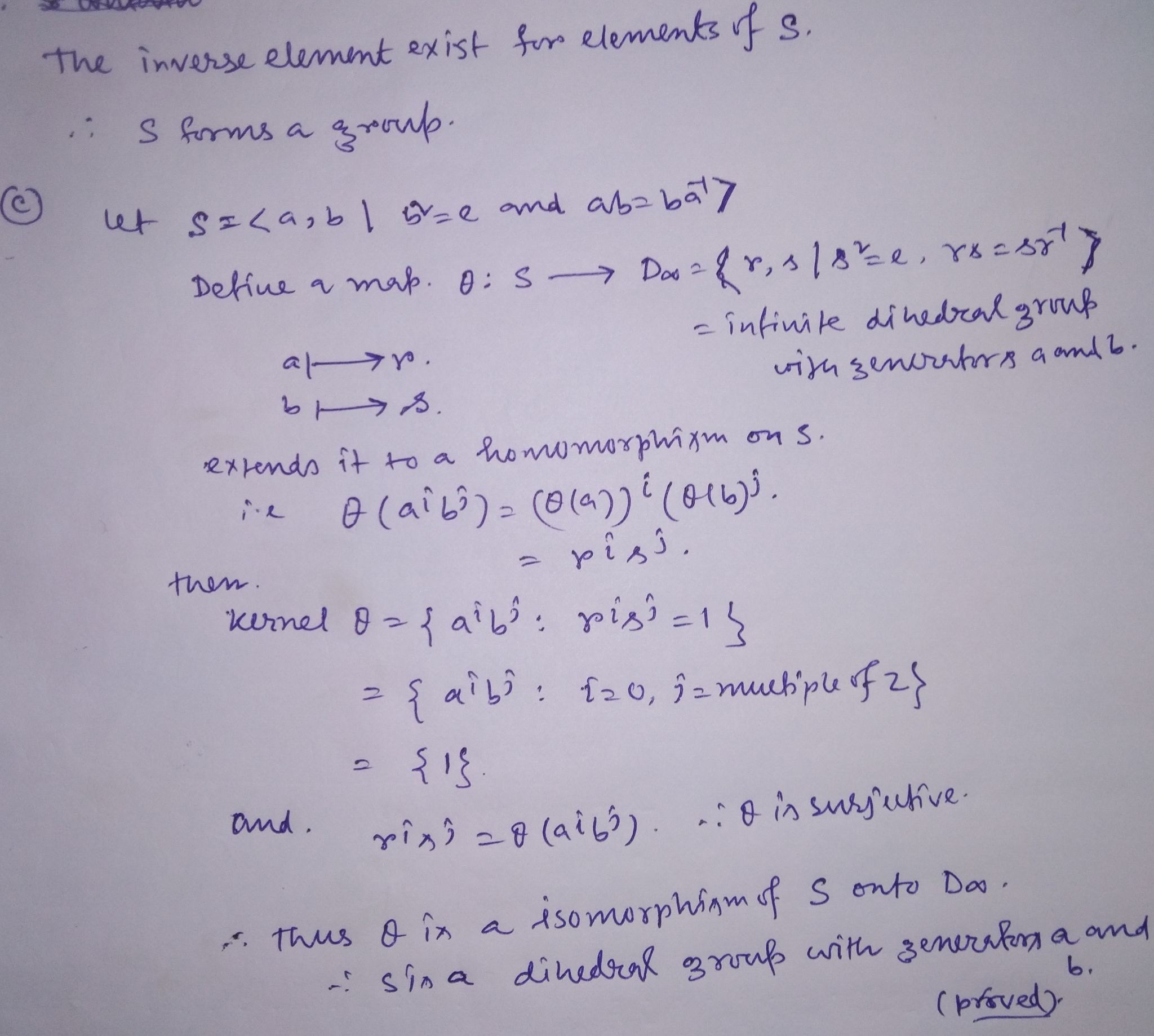##### Add Answer of: Let a and b be elements of a group G such that b has order 2...
Similar Homework Help Questions
• ### 1. Suppose a and b are elements of a group G. Prove, by induction, (bab−1)n = banb−1 . Hence prove that if a has order m, then bab−1 also has order m. Deduce from question (#1) that in any group ab an...

1. Suppose a and b are elements of a group G. Prove, by induction, (bab−1)n = banb−1 . Hence prove that if a has order m, then bab−1 also has order m. Deduce from question (#1) that in any group ab and ba have the same order (you may assume ab has finite order). The assertion in Question (#1) can be generalized to an assertion about isomorphisms. State and prove it.

• ### 1. Let a and b be elements of a group . Prove that ab and ba...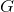1. Let a and b be elements of a group . Prove that ab and ba have the same order. 2. Show by example that the product of elements of nite order in a group need not have nite order. What if the group is abelian?

• ### a) Show that the positive rationals form a group under the operation a ∗ b =...

a) Show that the positive rationals form a group under the operation a ∗ b = ½ ab. b) Let G be a group, and suppose the elements g and h of G don’t commute. (i) Show that g doesn’t commute with gh. (ii) Find another element of G, distinct from h and gh, that doesn’t commute with g . c) Let G be a group, and let g be in G. (i) Prove that g and g^−1 have the...

• ### Group Theory 2

Let a and b be elements of a group G. Show that if ab has finite order n, then ba also has order n.

• ### Let D4 be dihedral group order 8. So D4={e, a, a^2, a^3, b, ab, a^2b, a^3b},   a^4 = e,...

Let D4 be dihedral group order 8. So D4={e, a, a^2, a^3, b, ab, a^2b, a^3b},   a^4 = e, b^2= e, ab=ba^3; A. FIND ALL THE COSETS OF THE SUBGROUP H= , list their elements. B. What is the index [D4 : H] C. DETERMINE IF H IS NORMAL

• ### Elementary Properties of Order

Let u, b and c be elements of a group G. Prove the following:-Ord(a)=ord(bab^-1)-the order ab is the same as the order of ba.

• ### dihedral group Dn

Let the dihedral group Dn be given by the elements a of order n and b of order 2, where ba=ba-1. Find the smallest subgroup ofDn that contains a2 and b. (hint: consider two cases, depending on whether n is odd or even.)

• ### Let G be a group, and let a ∈ G. Let φa : G −→ G...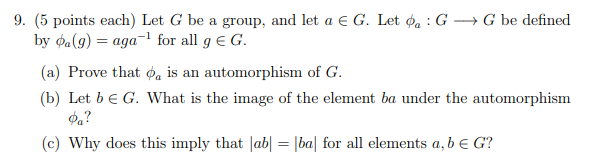Let G be a group, and let a ∈ G. Let φa : G −→ G be defined by φa(g) = aga−1 for all g ∈ G. (a) Prove that φa is an automorphism of G. (b) Let b ∈ G. What is the image of the element ba under the automorphism φa? (c) Why does this imply that |ab| = |ba| for all elements a, b ∈ G? 9. (5 points each) Let G be a group, and let...

• ### please look at red line please explain why P is normal thanks Proposition 6.4. There are (up to isomorphism) exactly three di groups of order 12: the dihedral group De, the alternating group A,...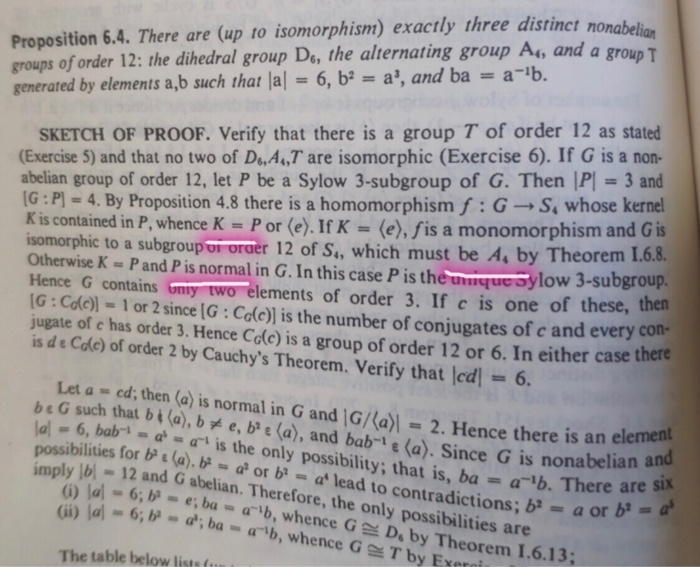please look at red line please explain why P is normal thanks Proposition 6.4. There are (up to isomorphism) exactly three di groups of order 12: the dihedral group De, the alternating group A, and a generated by elements a,b such that lal 6, b a', and ba a-b. stinct nonabelian SKETCH OF PROOF. Verify that there is a group T of order 12 as stated (Exercise 5) and that no two of Di,A,T are isomorphic (Exercise 6). If G...

• ### (5 points each) Let G be a group, and let a € G. Let da: G+...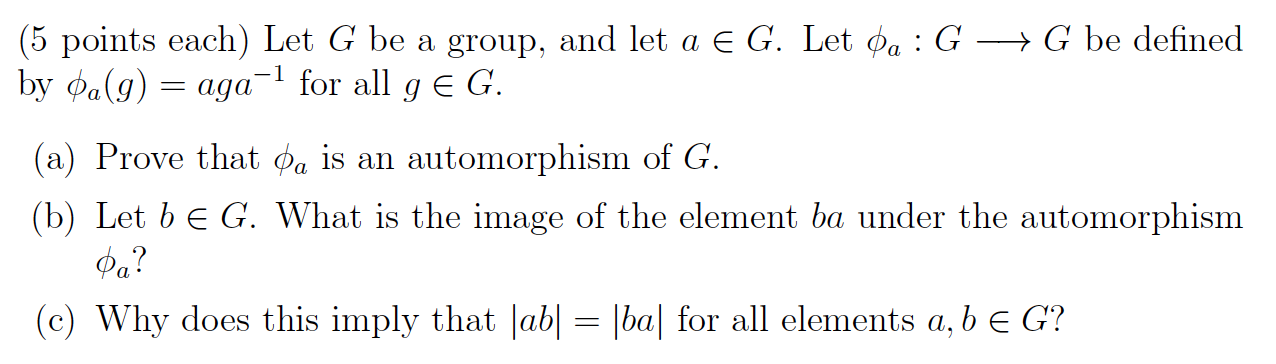(5 points each) Let G be a group, and let a € G. Let da: G+ G be defined by @a(g) = aga-l for all g E G. (a) Prove that Pa is an automorphism of G. (b) Let b E G. What is the image of the element ba under the automorphism ..? (c) Why does this imply that |ab| = |ba| for all elements a, b E G?

Free Homework App